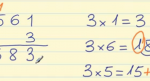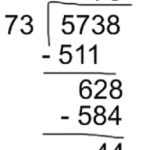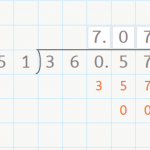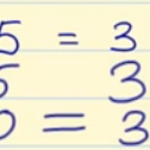Smartick is a fun way to learn math!# I want to learn about: Operations & Algebraic Thinking

Learn operations and algebraic thinking in elementary with Smartick. Exercises, theory, tutorials, and teaching resources about operations and algebraic thinking in elementary aged children. Here you will find posts about operations and algebraic thinking to make learning mathematics easier and more fun.

Jun24

## How to Multiply by a One-Digit NumberThis post will teach you how to multiply any number by a one-digit number. Multiplication Terms The numbers that are multiplied are called FACTORS and the answer is called the PRODUCT. The FACTORS are written on top of each other. Normally, the bigger number is written on the top, and it’s called the MULTIPLICAND and […]

Jun16

## How to Solve Double Digit DivisionIn today’s post we are going to explain how to solve double digit division. Before beginning to learn how to solve double digit division, it is important that you become familiar with these terms, because we will use them later. Dividend: the number that is being divided. Divisor: the number by which the dividend is […]

Jun10

## Dividing a Decimal by a Whole NumberDividing a Decimal by a Whole Number Hello everyone! In this week’s post, we are going to talk about dividing a decimal by a whole number. You already know how to divide a whole number by a whole number by now, right? If not, check out our posts about dividing by a one-digit number here, […]

Jun09

## Properties of MultiplicationThe properties of multiplication are distributive, commutative, associative, removing a common factor and the neutral element. We are dedicating this post to examining the properties of multiplication, which are the following: Distributive property: The multiplication of a number by a sum is equal to the sum of the multiplications of this number by each one […]